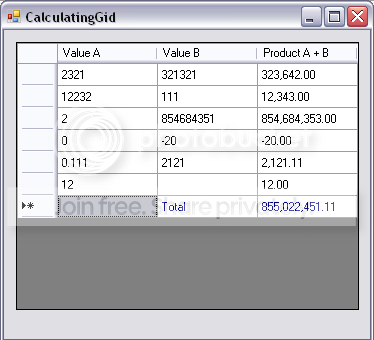#DataGridView Selected cells sum total• ### Question

•I have DataGridView1 where there is numeric data present. I want the sum of selected cells of DataGridView1 in my TextBox1 just like MS-Excell. How can i do this?
LuxCoder
Tuesday, March 9, 2010 9:56 AM

•If you want to do like excel then you can ignore string value.
Do you allow integer only or decimal as well, change Integer.TryParse if you allow other value types

``` Dim i As Integer = 0
Dim Sum = DataGridView1.SelectedCells.Cast(Of DataGridViewCell).Where(Function(f) Integer.TryParse(f.Value, i)).Sum(Function(f) f.Value)
TextBox1.Text = Sum.ToString```

Or if you want to stop user when they do not type integer then
```Dim i As Integer = 0
If DataGridView1.SelectedCells.Cast(Of DataGridViewCell).Any(Function(f) Not Integer.TryParse(f.Value, i)) Then
'error here
Else
Dim Sum = DataGridView1.SelectedCells.Cast(Of DataGridViewCell).Sum(Function(f) f.Value)
TextBox1.Text = Sum.ToString
End If
```

Arjun Paudel
• Proposed as answer by Tuesday, March 9, 2010 1:59 PM
• Marked as answer by Wednesday, March 10, 2010 6:08 AM
Tuesday, March 9, 2010 1:37 PM
•Here Again Linq :)

``` Dim Sum = DataGridView1.SelectedCells.Cast(Of DataGridViewCell).Sum(Function(f) f.Value)
TextBox1.Text = Sum.ToString```
In above example, you are taking selected cells and casting them to DatagridViewCell and using Extension method sum to sum them up.

Sorry following shows C# examples on MSDN not sure if that exists for VB

Arjun Paudel
• Marked as answer by Tuesday, March 9, 2010 11:08 AM
Tuesday, March 9, 2010 10:48 AM

### All replies

•That depends completely what is the datasource of your DataGridView

If it is a datatable then it is to do with the datatable compute,

if it is a generic list then you can use Linq
Success
Cor
Tuesday, March 9, 2010 10:02 AM
•Dear Luxcoder,

ScreenshotIt demonstrates how to create a calculating grid. You'd have to make a few adjustments to have it summarize the selected fields, but it should get you started. Also read through this thread, I think it will be usefull.

Hope this helps!

Cheers,

John

Tuesday, March 9, 2010 10:28 AM
•Here Again Linq :)

``` Dim Sum = DataGridView1.SelectedCells.Cast(Of DataGridViewCell).Sum(Function(f) f.Value)
TextBox1.Text = Sum.ToString```
In above example, you are taking selected cells and casting them to DatagridViewCell and using Extension method sum to sum them up.

Sorry following shows C# examples on MSDN not sure if that exists for VB

Arjun Paudel
• Marked as answer by Tuesday, March 9, 2010 11:08 AM
Tuesday, March 9, 2010 10:48 AM
•I am using the following code to determine integer datatype but it is giving me error if i select a string datatype cell. How should i validate this?

```Try
If WeekPayDataGridView.SelectedCells.GetType Is Type.GetType("System.Integer") Then
Dim Sum = WeekPayDataGridView.SelectedCells.Cast(Of DataGridViewCell).Sum(Function(f) f.Value)
ToolStripLabel1.Text = Sum.ToString
End If
Catch ex As Exception
MsgBox(ex.ToString, MsgBoxStyle.Exclamation)
End Try```
LuxCoder
Tuesday, March 9, 2010 1:23 PM
•If you want to do like excel then you can ignore string value.
Do you allow integer only or decimal as well, change Integer.TryParse if you allow other value types

``` Dim i As Integer = 0
Dim Sum = DataGridView1.SelectedCells.Cast(Of DataGridViewCell).Where(Function(f) Integer.TryParse(f.Value, i)).Sum(Function(f) f.Value)
TextBox1.Text = Sum.ToString```

Or if you want to stop user when they do not type integer then
```Dim i As Integer = 0
If DataGridView1.SelectedCells.Cast(Of DataGridViewCell).Any(Function(f) Not Integer.TryParse(f.Value, i)) Then
'error here
Else
Dim Sum = DataGridView1.SelectedCells.Cast(Of DataGridViewCell).Sum(Function(f) f.Value)
TextBox1.Text = Sum.ToString
End If
```

Arjun Paudel
• Proposed as answer by Tuesday, March 9, 2010 1:59 PM
• Marked as answer by Wednesday, March 10, 2010 6:08 AM
Tuesday, March 9, 2010 1:37 PM
•no fun helping you, no points to earn.... :p

anyways, this should get you started
```  'Usage: SumSelectedCells(Me.MyDataGridView)

Public Function SumSelectedCells(ByVal DataGridView As DataGridView) As Double
Try
Dim Cell As DataGridViewCell
Dim Total As Double

For Each Cell In DataGridView.SelectedCells
If CastAsNumber(Cell.Value) = True Then
Total = Total + Cell.Value
End If
Next
Catch ex As Exception
Debug.Print(ex.ToString)
End Try
End Function

Public Function CastAsNumber(ByVal CellValue As String) As Boolean
Try
Dim value As Double
value = CDbl(CellValue)
Return True
Catch ex As Exception
Return False
End Try
End Function```
• Proposed as answer by Tuesday, March 9, 2010 1:59 PM
Tuesday, March 9, 2010 1:46 PM
•Could you please tell me why it does not return decimal values only interger.

I need it, to give me decimal values.

Dim i As Double = 0
Dim Sum As Double = DataGridView2.SelectedCells.Cast(Of DataGridViewCell).Where(Function(f) Double.TryParse(f.Value, i)).Sum(Function(f) f.Value)
TextBox1.Text = Sum.ToString